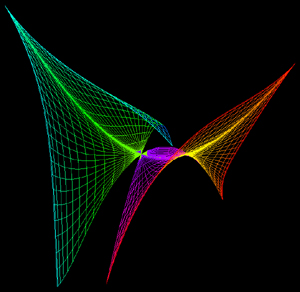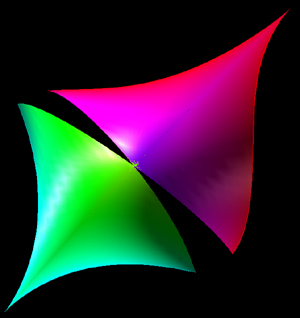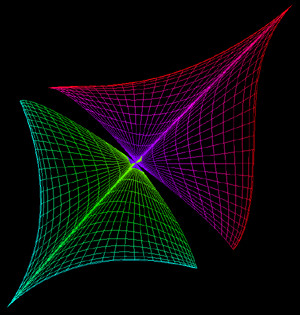# Tractrix Based Minimal Surface

By Roger Bagula
Graphics by Paul Bourke

January 2003

x = cos(u) (v - tanh(v))
y = cos(u) / cosh(v)
z = x2 - y2 + 2 x y tanh2(u)
-pi <= u <= pi, -pi <= v <= pi